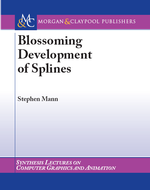# Errata for A Blossoming Approach to SplinesLast Updated: March 12, 2020

Book available from amazon and as an ebook.

• pg 18 - Exercises 2 and 3. The polynomial should be
F(x) = x3+x2+x+1
• pg 22 - Example 2.4 should say
• and we construct f(u̅1, u̅2, u̅3) by blossoming each term:
• pg 26 - first sentence of second paragraph is missing the period at the end of the sentence.
• pg 29 - The proof is incorrect in the last step when it cancels an f* term with a g* term. A double inductive proof is required, on i (the derivative) and l (the l in the proof).
• pg 32 - Figure 2.9. Second row from bottom, last node should be labled f*(1̅,δ,δ)
• pg 40 - Both figures:
• left f(t_1,t_2,t) should be f(t_2,t,t)
• right f(t_1,t_2,t) should be f(t_3,t,t)
• pg 50 - Knot vector in paragraph about the Oslo algorithm should not have a minus sign in front of u_4.
• pg 50 - In the paragarph about the Oslo algorithm, only the left edge gives us control points; the right edge does not.
• pg 52 - Ni0(u) should be defined to be 1 if ui-1<= u < u (i.e., there should be "<=" on one side of u and "<" on the other side).
• pg 55, 3rd line of first paragraph, "we see the a single..." should be "we see the single..."
• pg 59, end of next to last paragraph. The interval should be
[tn-1, tL+n-1]
• pg 65 - two summations at bottom of page - neither one should have an n on top of the sum.
• pg 75 - Section 4.1.8, first line of pseudo-code for first algorithm should be
```		for i:=0 to n-1 do
```
• pg 87 - the sum in the display equation should be to s,t not n,m

Stephen Mann
smann@uwaterloo.ca
University of Waterloo | School of Computer Science | 200 University Ave. W. | Waterloo, Ontario Canada | N2L 3G1 | 519.888.4567 | www.cgl.uwaterloo.ca/~smann/